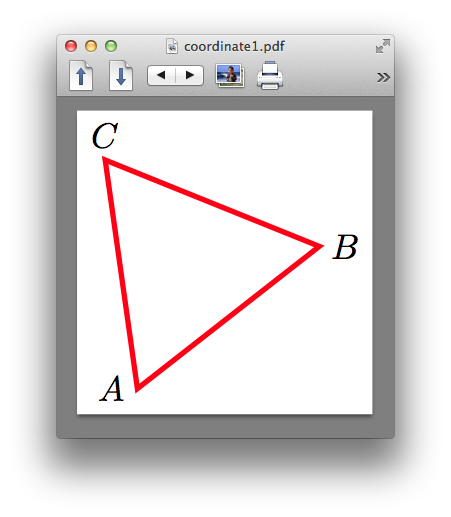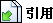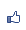TiKz 座標，運算
2015/04/02 21:07:07瀏覽1457｜回應0｜推薦0

\coordinate (A) at (1,2);

\draw (0,0)--(A);

\coordinate (B) at (30:1cm);

\coordinate (C) at (1,{sqrt(3)});

\coordinate (D) at (360/6 *3:1);

\usetikzlibrary{calc}

calc支援了對座標值直接的加減和乘上系數的運算，在小號內的雙中間，來運算，例如二點的中點：

\coordinate (D) at ($(A)!.5!(B)$);

B繞A旋轉30度的座標：

\coordinate(E) at ($(A)!1!30:(B)$); 第一個值是長度比例值，第二值是角度值。

\coordinate (D) at ($(A)!(C)!(B)$);

\coordinate [label=left:{$A$}] (A) at (1,0);

\node 是帶有座標的物體，該物體可能是形狀或文字⋯

\node (A) at (1,0){};

(A)就是一個在(1,0)的一個無形的物體，它可以由很多參數操作，做出很多的效果。如果要畫出來：

\node [draw] (A) at (1,0){};

\documentclass{ctexart}

\usepackage{tikz}

\usetikzlibrary{calc}

\begin{document}

\begin{tikzpicture}
\coordinate [label=left:{$A$}] (A) at (1+0.1*rand,.5+0.1*rand);
\coordinate [label=right:{$B$}] (B) at (3+0.1*rand, 2+0.1*rand);
\coordinate [label=above:{$C$}] (C) at ($(A)!.5!(B)!{sqrt(3)}!90:(B)$);
\draw[red, ultra thick] (A)--(B)--(C)--cycle;
\end{tikzpicture}
\end{document}( 知識學習科學百科 )引用網址：https://classic-blog.udn.com/article/trackback.jsp?uid=paraquat&aid=21951723